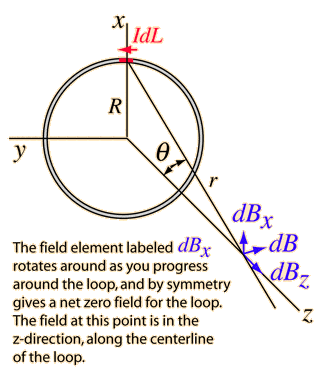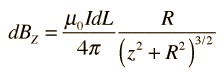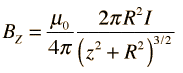# Magnetic field inside a Solenoid

## Homework Statement

Can I derive an equation for magnetic field inside a solenoid using the formula for magnetic field on the axis of a current carrying coil?

## Homework Equations

B = μI/2r ( Magnetic field at the centre of a current carrying coil)

## The Attempt at a Solution

B = μI/2r
Let the number of turns per unit length of the solenoid be 'n' and its length be 'a'
So,
B = μnaI/2r
Which is definitely not equal to μnI (Calculated using Ampere's Circuital Law)
What's wrong?[/B]

ehild
Homework Helper

## Homework Statement

Can I derive an equation for magnetic field inside a solenoid using the formula for magnetic field on the axis of a current carrying coil?

## Homework Equations

B = μI/2r ( Magnetic field at the centre of a current carrying coil)
The formula refers to the magnetic field of an infinitely long straight current-carrying wire, at distance r from the wire. It is not valid in the centre of a loop.

rude man
Homework Helper
Gold Member
Plus, the formula for the mag field around an infinite, current-carrying wire is B = μI/2πr, not what you wrote.

Well, my teacher has been using that formula...The application of the Biot Savart Law on the centerline of a current loop involves integrating the z-component.The symmetry is such that all the terms in this element are constant except the distance element dL , which when integrated just gives the circumference of the circle. The magnetic field is thenSo, if we apply the same,
z = 0 (At the centre of the loop)
So, B = μI/2R
Isn't it correct??

ehild
Homework Helper
It is correct for the magnetic field at the centre of a single loop. If you have a coil, all loops have their magnetic field inside the other loops. You should use the formula for B(z) and sum (integrate) the contributions of all loops.

rude man
Homework Helper
Gold Member
Isn't it correct??
Yes, but tha's for a single loop, using Biot-Savart. Not the thing for here..

Rather than integrate per post #5 my hint is to form a closed loop going thru the entire solenoid middle and closing outside the solenoid. You can now apply Ampere's law to get B.
Hint: contributions to the integral outside the loop may be ignored.

•Prashasti